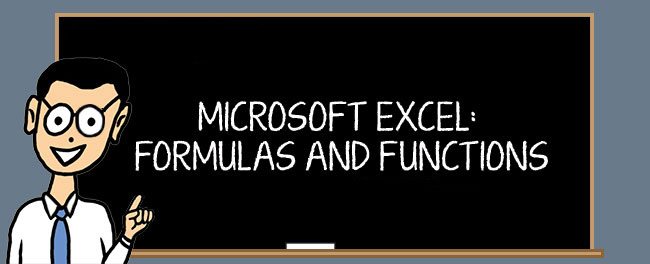# Excel Functions and Formulas Training

Do you need that extra boost to develop more complex functions and formulas? In this class you will learn advanced Excel formulas and functions to bring your spreadsheets to the next level. Learn how to develop more complex formulas and learn the power of arrays. Learn formulas to help you automate your spreadsheets to simplify them for easier use for others, as well as yourself.

## Training Goal

Bring your brains for this one! Students will leave this class with a solid understanding of advanced Excel formulas and functions that can help with their more complicated projects. This course takes an intense look at complex formulas, as well as how to nest several different functions. Students will learn how to create dynamic drop-down lists to automate their spreadsheets for others to use. Arrays will also be applied to expand formulas to wider uses.

## PreRequisites

Students should enter this course with an understanding of Excel Intermediate and Advanced topics, including basic formulas, IF Statements, and basic Lookup formulas. This is an intense one day course, focusing on learning about functions and formulas.

## Topics Covered

• Workbook Efficiency Techniques
• Logical and Information Functions
• Nesting IF statements and Dates Functions
• AND Functions
• Text and Lookup Functions
• Find
• Len
• Concatenate
• Lookup Functions
• VLOOKUP
• HLOOKUP
• ISTEXT Functions
• ISODD Functions
• ISBLANK Functions
• Array Functions with Transpose
• Array Functions and SUM used with IF statements
• Building Intelligence
• Complex Nested IF Functions
• Using Functions to Create Dynamic Dropdown Lists
• VLOOKUP drop-down lists
• IF and VLOOKUP combination functions
• Indirect and VLOOKUP combination functions
• Offset drop-down lists
• Filtered Columns
• ISTEXT function
• ROW function
• ISTEXT and ROW nested with IF functions
• SMALL function
• INDEX function
• IFERROR function
• ARRAY function
• Validation Lists
• Removing Blanks
• No Blanks

In this advanced Excel formulas and functions course, you will learn applications for a large variety of functions by themselves and combined, enabling you to become a powerhouse in the world of spreadsheets.